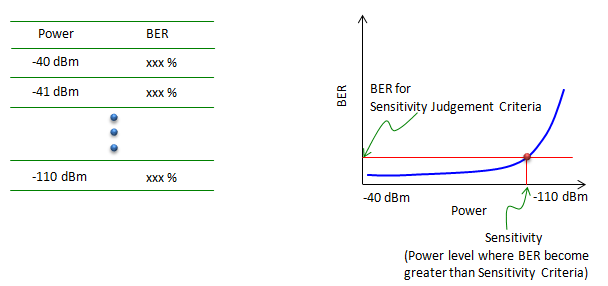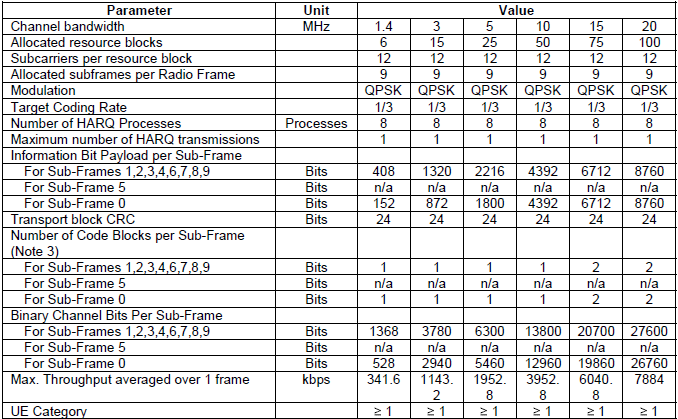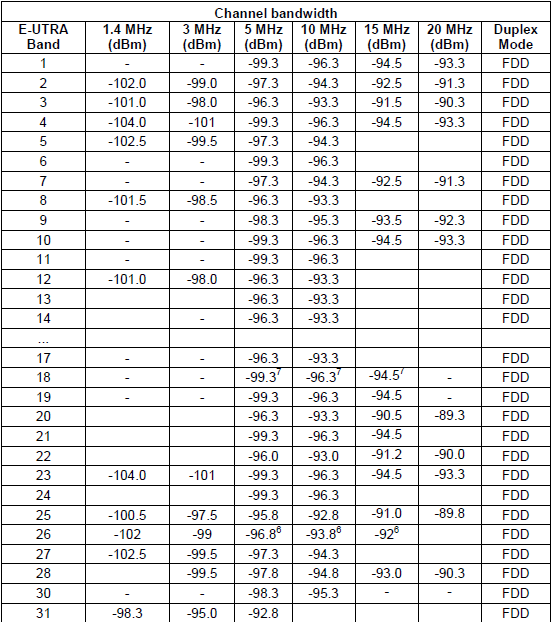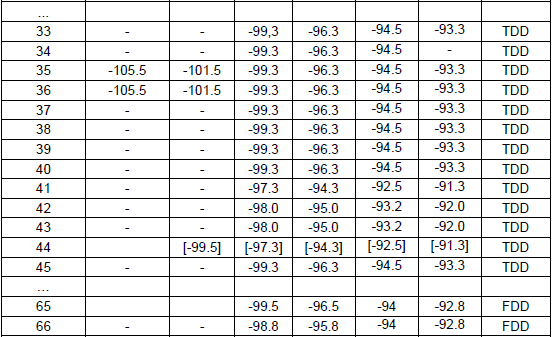RF - Sensitivity                                     Home : www.sharetechnote.com Sensitivity is an indicator showing how well a device receive the signal and decode it within a 'satisfactory error rate'. (How low the error rate should be for 'satisfactory error rate' is normally defined by a specification of each application). Sensitivity is represented in a power level (e.g, -100 dBm). So the interpretation of the sensitivity value goes like this. Let's assume that you (or specification) set BER 1 % to be the level of 'satisfactory error rate'. and assume that the sensitivity of your device is measured to be -100 dBm. This means that the reciever power level down to -100 dBm, BER measured by the device is lower than 1% and if the reciever power gets lower than -100 dBm, the BER gets larger than 1%.  Here goes another case. Let's assume that you have two device (Device A and B). Device A has the sensitivity of -100 dBm and device B has the sensitivity of -110 dBm. In this case, we can say "Device B has better sensitivity and better reception capability".     How to measure ?   Now let's think about how to measure the sensitivity of a device. There are a couple of different ways of measuring the sensitivity, but the most common method is as illustrated below. In both case, we use a specific test equipment which can communicate with the specific DUT with same protocol. For example, if the DUT is a bluetooth device, we use a test equipment which is working in bluetooth protocol (e.g, Anritsu MT8852) and if the DUT is WCDMA device, the test equipment which is supporting WCDMA protocol (e.g, NodeB simulator like Anritsu MT8820 or R&S CMW 500)   There can be two different setups to measure sensitivity using these equipments. In the setup shown on the left, the test equipment transmit the test signal (a known bit sequence) and DUT compares the received bit sequence with the bit sequence generated internally and calculate BER (Bit Error Rate). In the setup shown on the right, the test equipment transmit a bit sequence and UE recieves and retransmit (loopback) the received sequence to the equipment, and the equipment compares the transmitted bit sequence and loopbacked bit sequence and calculate the BER.Usually we cannot figure out the sensitivity in a single step and have to repeat the measurement procedure described above multiple steps with different power level. For example, you can measure BER as explained above with power level -40 dBm and measure BER and write down the value. Next, do the same measurement at power level -41 dBm. Repeat this process all to way down to -110 dBm. If you plot the test result, you would see a plot as shown on the right. In this plot, you can find a point (Power level) where BER gets greater than the specified criteria. That power is called 'Sensitivity'.Estimation of Sensitivity / Factors affecting the Sensitivity   The method described above would be the most accurate way to do the sensitivity measurement, but to test in this way you must be ready with the following two items i) Equipment which support protocol stack for the DUT ii) Device should have been fully implemented in terms of baseband, both TX and RX path. Is there any other way to figure out the sensitivity without meeting the criteria listed above(i.e, without doing the real measurement) ? It is impossible to get the pricise sensitivity value for a specific device without performing the real measurement, but there is a way to roughly estimate the value using a formula as shown below. (This equation uses only RF level parameters, like NF(Noise Figure) and System BW and SNR(Signal To Noise Ratio), you can apply this equation to not only a system level but also component level (e.g, Amplifier))There are several major factors that influence on Sensitivity as listed below. The effect of the item i),ii),iii) can be seen in the equation shown above. In recent high end communication technology (e.g, WCDMA, LTE etc), many of techniques are added to improve sensitivity (i.e, decrease error rate and increase communication reliability). Most common techniques adopted in those high end communication are Adaptive Modulation and Channel Coding/Error Correction. As a result, item iv) and v) became an important factors for Sensitivity performance. i) System Bandwidth ii) Noise Figure iii) SNR iv) Modulation Scheme v) Channel Coding / Error Correction Method One of the examples showing the impact of item iii), iv), v) is shown below (Ref ). As you see, this graph does not directly show about Sensitivity, but the Sensitivity is directly related to BER or BLER. You may say High BLER indicate poor sensitivity performance and Low BLER indicate good sensitivity performance. This example is for LTE. The MCS value in LTE defines a specific modulation scheme and Code Rate (i.e, parameter of Channel Coding/Error Correction). As you see here, Low MCS shows lower BLER if SNR is same. It means Low MCS will show good sensitivity performance than higher MCS.Conditions for Sensitivity Measurement   As mentioned above, there are many factors that influence the Sensitivity. So when you do sensitivity measurement, you have to clearly define the conditions for the measurement especially when you want to use the measured value for quality control or performance comparision with other devices. If you are working on well known/widely used communication system, the specific measurement conditions for Sensitity are defined in the industry standard /specification. For example, if you are working with Bluetooth, you may find those condition from Bluetooth SIG specification. If you are working on WLAN, you may find the condition from IEEE specification. In case of LTE, the conditions are defined in 3GPP 36.521.   Following is an example of Sensitivity measurement condition for LTE based on 3GPP 36.521 - 7.3 Reference sensitivity level.   The condition is specified as in 36.521-Table A.3.2-1. If I summarize the highlights, it is as follows No Interference (very good SNR) Number of RB : Maximum Number of RBs allowed for the specified System Bandwidth MCS : 4  // Sensitivity is measured with very robust demodulate condition. The expected sensitity power (passing critieria) is defined in 36.521 - Table 7.3.5-1 for single Rx antenna case and Table 7.3_1.5-1 for 4 Rx antenna case. As you see, the passing criteria is slightly different for each system Bandwidth and with operating band(carrier frequency).   < 36.521 - Table A.3.2-1: Fixed Reference Channel for Receiver Requirements (FDD) >< 36.521 - Table 7.3.5-1: Reference sensitivity QPSK P_PREFSENS >< 36.521 - Table 7.3_1.5-1: Reference sensitivity QPSK P_PREFSENS >Reference :    NISTIR 7986 - LTE Physical Layer Performance Analysis by Wen-Bin Yang, Michael Souryal